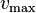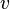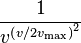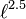# Propulsion and drag

## Propulsion

For a top speed$v_\max$ and forward velocity of$v$ (always at least 1 m/s), thrust is multiplied by$\frac{1}{v^{\left( v / 2 v_\max \right)^2}}$

## Linear drag

### Cross section

Cross section is determined by the frontmost faces of the construct as listed in Blocks.

Orientation does not matter, only the shape of the block.

### Length friction

Length friction is determined by the cross sectional area on the four sides of the construct. In air, each m2 of such contributes 0.001 length friction regardless of shape. The length friction in water is larger but it does not seem to be computed the same way.

### Drag force

The drag force is equal to$v^2 \cdot \left( \text{cross section} + \text{length friction} \right)$

Linear drag is always applied at the center of gravity, and thus never produces a torque.

Drag is 20 times as large in water.

## Angular drag

The angular drag coefficients for a beam of length$\ell$ are proportional to$\ell^{2.5}$. Therefore, it is conjectured that each face contributes angular drag coefficient proportional to its distance perpendicular to the axis under question (which passes through the center of gravity), to the 1.5 power.

The displayed angular drag has a minimum of 0.2. It is currently unknown what units this is in, or whether it represents angular acceleration, proportion of angular velocity lost per time, or something else.

Known facts:

• The displayed angular drag is increased by both angular velocity and by the angular drag coefficient.
• The displayed angular drag is sublinear with respect to the applied external torque.